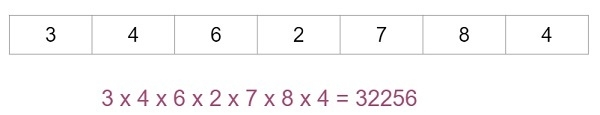# C++ program for multiplication of array elements

Given with an array of integer elements and the task is to multiply the elements of an array and display it.

## Example

Input-: arr[]={1,2,3,4,5,6,7}
Output-: 1 x 2 x 3 x 4 x 5 x 6 x 7 = 5040
Input-: arr[]={3, 4,6, 2, 7, 8, 4}
Output-: 3 x 4 x 6 x 2 x 7 x 8 x 4 = 32256

Approach used in the below program is as follows

• Initialize temporary variable to store the final result with 1
• Start loop from 0 to n where n is the size of an array
• Keep multiplying the value of temp with arr[i] for final result
• Display the value of temp which will be resultant value

Given below is the example of multiplying the inputs and generating required output## Algorithm

Start
Step 1-> Declare function for multiplication of array elements
int multiply(int arr[], int len)
set int i,temp=1
Loop For i=0 and i<len and i++
Set temp=temp*arr[i]
End
return temp
step 2-> In main()
Declare int arr[]={1,2,3,4,5,6,7}
Set int len=sizeof(arr)/sizeof(arr)
Set int value = multiply(arr,len)
Print value
Stop

## Example

Live Demo

#include<stdio.h>
//function for multiplication
int multiply(int arr[], int len) {
int i,temp=1;
for(i=0;i<len;i++) {
temp=temp*arr[i];
}
return temp;
}
int main() {
int arr[]={1,2,3,4,5,6,7};
int len=sizeof(arr)/sizeof(arr);
int value = multiply(arr,len);
printf("value of array elements after multiplication : %d",value);
return 0;
}

## Output

IF WE RUN THE ABOVE CODE IT WILL GENERATE FOLLOWING OUTPUT

value of array elements after multiplication : 5040